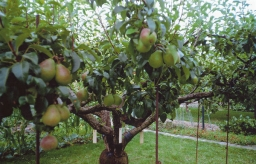# Afforestation 24341

During the afforestation, 2,950 saplings were planted in three days. On the second day, 25% more saplings were planted than on the first day, and on the third day, 15% more than on the second day. How many saplings were planted on each day?

a =  800
b =  1000
c =  1150

### Step-by-step explanation:

a+b+c=2950
b = a+0.25 a
c = b+0.15 b

a+b+c=2950
b = a+0.25·a
c = b+0.15·b

a+b+c = 2950
1.25a-b = 0
1.15b-c = 0

Pivot: Row 1 ↔ Row 2
1.25a-b = 0
a+b+c = 2950
1.15b-c = 0

Row 2 - 1/1.25 · Row 1 → Row 2
1.25a-b = 0
1.8b+c = 2950
1.15b-c = 0

Row 3 - 1.15/1.8 · Row 2 → Row 3
1.25a-b = 0
1.8b+c = 2950
-1.639c = -1884.722

c = -1884.72222222/-1.63888889 = 1150
b = 2950-c/1.8 = 2950-1150/1.8 = 1000
a = 0+b/1.25 = 0+1000/1.25 = 800

a = 800
b = 1000
c = 1150

Our linear equations calculator calculates it.Did you find an error or inaccuracy? Feel free to write us. Thank you!

Tips for related online calculators